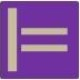## Models: Correct, Complete, Consistent, Unambiguous

How do you judge how ‘good’ or ‘bad’ a model is? I mean models like we use them in software requirements specification or business analysis. On this, one can find criteria in literature like BABOK, Wikipedia, IEEE, research papers, or textbooks. However, for some reason these criteria sets are quite different in each case. Since I find the status quo a ‘bit’ confusing, subsequently I’ve tried to get the things a little straighter, starting with the ‘big four’ quality characteristics of software requirements specifications, these are: Correct, Complete, Consistent, and Unambiguous. (wording after IEEE 830)In the figure, let
C be the set of all solutions accepted by the customer,
M the set of all solutions according to the requirements model, and
P the set of all possible solutions (solely in a logical, not in a technical sense).

One may think of the requirements model as the edge of M, that restricts the solutions to M.

Now we can think of the characteristics in the following way:
Correct: No requirement in the model is wrong and thus every solution accepted by the customer (in C) is in accord with the model (in M), i.e. C is a subset of M, as in diagram (correct). Notice, thus correct requirements can lead to incorrect solutions.
Complete: No requirement in the model is missing and thus every solution according to the model (in M) is also accepted by the customer (in C), i.e. M is a subset of C, as in diagram (complete). Notice, thus complete requirements can describe the solution set incompletely.
Consistent: There is at least one possible solution and thus the modelled and the possible solutions (M and P) have an intersection, as in diagram (consistent).
Unambiguous: There is at most one possible solution and thus the modelled and the possible solutions (M and P) might have no intersection, as in diagram (unambiguous).

Notice, that correct and complete means, that M and C are congruent. Also Consistent and Unambiguous means, that M and P have exactly one point in common, and thus all four together imply, that the model describes a unique accepted and possible solution.

So long
|=## About modelpractice

Modeling Theory and Abstraction Awareness in strive for scientific rigour and relevance to information systems engineering.
This entry was posted in Mathematics, Requirements and tagged , , , , , , , , , , , , , , , , , , , , , . Bookmark the permalink.